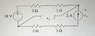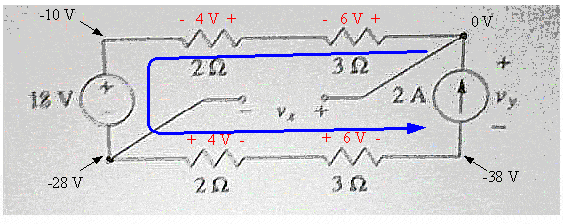# Solving a simle circuit with a voltage and current source

• Engineering
• Jarvis323
In summary, the current source must be open circuited in order for the current to flow in the correct direction.

#### Jarvis323

Attached is the model.

Find Vx and Vy.

## The Attempt at a Solution

The total resistance is 10 ohms, and the circuit elements are all in series. So 2 Amps of current is flowing in the direction of the arrow in the diagram across all elements, Vtotalsrc = (to the voltage from the current source and the voltage from the voltage source) must be 20 so that I = V/R = 2 Amps.

The way that I looked at it, Vy must be -2 volts. This way, The voltage from the current source reduces the overall current by -2 volts, putting it at 20 volts, and the current (flowing from high potential to low potential) is in the direction of the arrow on the current source. This would put Vx at 8 volts.

Anther student has the book that the problem is out of, and the solution it gives is Vy = 38 volts. Then, I = (18 - 38) / 10 = -2A. In other words, you get 2 Amps flowing in the opposite direction of the arrow in the picture.

Most people were trying to solve this problem, by knowing that there is a 10V voltage change across both the lower two resistors and upper two. But you need to know the direction of the voltage drop/gain. So you have to draw a few plus signs. The choices you make then dictate if you get Vy = 38, or Vy = -2. So if you went this approach, how do you determine the direction of the voltage drop across the resistors? If you choose to follow that the voltage drop is in the direction of the current which I would have done, then you get Vy = -2V.

Any thoughts, or confirmation that the book is wrong would be helpful. Thank you.

#### Attachments

•circuit.jpg
21.2 KB · Views: 458
You cannot, by definition, have the current through the current source flowing in the wrong direction. I get a different answer from the book AND from you.

•1 person
phinds said:
You cannot, by definition, have the current through the current source flowing in the wrong direction. I get a different answer from the book AND from you.

You can if the voltage is negative, because then it's equivalent to going the opposite direction a positive amount. ie. (+ 2V - == - (-2V) +). And if you have any other values then Vy = 38, or Vy = -2V, then it is impossible to have 2 amps of current in either direction. I.E. if Vy is negative, then the current source is flowing in the direction of the voltage drop across the current source.

Nope. Phinds is correct. An ideal current source will produce its rated current in its specified direction (arrow inside the symbol) no matter what, even if it must destroy the rest of the circuit to do it. It will produce any amount of voltage required to accomplish its mission.

Of course this means that an open-circuited ideal current source would be something to be fearedSimilarly, an ideal voltage source will produce any amount of current required to maintain its potential difference. Don't short circuit an ideal voltage source!

•1 person
gneill said:
Nope. Phinds is correct. An ideal current source will produce its rated current in its specified direction (arrow inside the symbol) no matter what, even if it must destroy the rest of the circuit to do it. It will produce any amount of voltage required to accomplish its mission.

Of course this means that an open-circuited ideal current source would be something to be fearedSimilarly, an ideal voltage source will produce any amount of current required to maintain its potential difference. Don't short circuit an ideal voltage source!

I guess I misinterpreted what he said. But this is the reason that I think my answer must be correct, because it does result in the current flowing in the correct direction. So the books answer can be ruled out. But how then can Phobos get a different answer? It must be Vy = -2V correct?

tAllan said:
I guess I misinterpreted what he said. But this is the reason that I think my answer must be correct, because it does result in the current flowing in the correct direction. So the books answer can be ruled out. But how then can Phobos get a different answer? It must be Vy = -2V correct?

The book's answer for Vy looks fine to me.

Do a KVL "walk" starting from the top of the current source (where you arbitrarily call that point "0 Volts" as a reference point). Keeping in mind the size and direction of the enforced current, add up the potential changes until you get to the bottom of the current source. The current source's potential must make up any difference between the value you've added up and 0 V.

•1 person
gneill said:
The book's answer for Vy looks fine to me.

[wrong calc deleted]

AH ... got it. I was reading the voltage source as 12V, not the 18V that it is actually labeled, so now I agree.

•1 person
gneill said:
The book's answer for Vy looks fine to me.

Do a KVL "walk" starting from the top of the current source (where you arbitrarily call that point "0 Volts" as a reference point). Keeping in mind the size and direction of the enforced current, add up the potential changes until you get to the bottom of the current source. The current source's potential must make up any difference between the value you've added up and 0 V.

That's true, but if you draw the polarity of the voltage across the resistors in the same direction as the current source, then you get Vy = -2V, and the KVL is also still satisfied, and you still satisfy V = IR.

The difference between the two solutions is which way the current is flowing. The direction of the current is given, so it must match up with the solution. So I guess where I am confused is how you can determine absolutely which solution is consistent with the given current direction.

So again the way I interpret it, you can get Vx, and then combine the two voltage sources into one, with a potential difference of 20V. And then of course 20V = 2A / 10 Ohms. You have 2A given as positive, so your answer must be consistent with that, and the resistance is positive, so your single voltage source as a combination of the the two must be +20V.

Because the polarities of the two voltage sources are opposite each other, you must take the difference, and maintain the polarity of the larger one.

So if you have 18 and -2, you get 20 in with one polarity, and if you have 18 and 38, then you get 20 with the opposite polarity. So I guess my question is, in order to be consistent with the direction of the current, do you want your overall source polarity to be aligned with Vy, or the 18 Volt source.

Is it the case that you alway align + with the tip of the arrow of the current source. And when you have a single voltage source, does the current always flow in the direction of the + end?

(- v +) ==> +I-->
or
(+ v -) ==> +I-->
?

Sorry if I am using too many words to explain y misunderstanding. Thanks for the help.

phinds said:
[wrong calc deleted]

ah ... Got it. I was reading the voltage source as 12v, not the 18v that it is actually labeled.

edit: ok

Here's your circuit with the potential changes indicated. The current direction is fixed in size and direction by the current source as discussed. That makes every potential change around the loop a drop if you walk around it counterclockwise. I've also indicated the potentials at various points of interest.Note that the current direction specifies the directions of the polarity changes across the resistors. There is no fudging that! Potential drops in the direction of current flow through a resistor unless the "resistor" is actually a special device that exhibits "negative resistance". No such beasts in use here!

#### Attachments

•1 person
Thanks. I should have realized that. That clears things up completely.